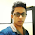• 8bit
• signed
• -128 to 127

### short

• 16bit
• signed
• -32768 to 32767

### int

• 32bit
• signed (since Java 8 it can be used to represent unsigned)
• -231 to 231-1

### long

• 64bit
• signed (since Java 8 it can be used to represent unsigned)
• -263 to 263-1

### float

• Single precision 32bit
• End with F or f

### double

• Double precision 64bit
• End with D or d

### boolean

• Only two values (true / false)

### char

• Single 16bit unicode charactor (UTF 16)
• '\u0000' to '\uffff' (0 to 65535)

Here you can try this program to understand about java data types.

```public class DataTypes {

public static void main(String[] args) {

byte b = 10;
short s = 5478;
int i = 9978456;
long l = 12547893533245625L;
float f = 3.142f;
double d = 1.0000000002125d;
char c1 = 'A';
char c2 = '\u0024';
String st = "This is String";

System.out.println("byte: " + b);
System.out.println("short: " + s);
System.out.println("int: " + i);
System.out.println("long: " + l);
System.out.println("float: " + f);
System.out.println("double: " + d);
System.out.println("char: " + c1);
System.out.println("char unicode: " + c2);
System.out.println("String: " + st);
}
}

```

### Upward casting

This method is implicit and reliable.
There is the way to convert.

int i = 10 ;
double d = i ;

```public class DataTypes {

public static void main(String[] args) {

int i = 100;
double d = i;
System.out.println(d);
}
}

```

### Downward casting

In this situating you can’t cast like upward method,If you try that way, it will give a compile time error.

double d = 3.14;
int I = d;

So you have to cast in this way, Now output is 3.

double d = 3.14;
int I = (int) d;

Your result is 3, double is floating point data type, but int is integer type.That is why you got the answer 3 instead of other one.

Try this code,

```public class DataTypes {

public static void main(String[] args) {

int i = 128;
byte b = (byte) i;

System.out.println(b);

}
}

```

In this program your output is -128,

You may confuse from this answer. Thing is that, the range of byte is -128 to 127. When it exceeds the limit, its wraparound. Then the next value after 127 is -128.

### Special escape characters

• \b - backspace
• \n - new line
• \t - tab
• \f - form feed
• \r - carriage return
• \" - double quote
• \' - single quote
• \\  - backslash

### Underscore character

• After Java SE 7, you can use underscore (_) character in numerical fields.
• This can be used to separate numbers into groups to read easy.
• But you can use it at the beginning or at the end of the number.
• You cannot put underscore just before or after the F, D or L character.
• It cannot be used before or after the decimal sign.

```public class DataTypes {

public static void main(String[] args) {

long num1 = 5642_1245_7845_5458L;
float num2 = 3.14_15F;
long num3 = 0xFF_AC_FA;

System.out.println(num1);
System.out.println(num2);
System.out.println(num3);

}
}

```

### Default values of data types

Try this code to check default values in java.

```public class DataTypes {

static byte b;
static short s;
static int i;
static long l;
static float f;
static double d;
static String str;
static boolean bool;

public static void main(String args[]) {

System.out.println("byte : " + b);
System.out.println("short : " + s);
System.out.println("int : " + i);
System.out.println("long : " + l);
System.out.println("float : " + f);
System.out.println("double : " + d);
System.out.println("String :" + str);
System.out.println("boolean : " + bool);
}
}

```

Data types in javaReviewed by Ravi Yasas on 9:28 PM Rating: 5

1.In your diagram displaying primitive data types shouldn't boolean and char be included, say under "nun numeric"??

1.I have corrected it bro, thankz much...

2.It's better to have your kind comments to improve this blog, I really forgot it when I create it. anyway Thanks ..